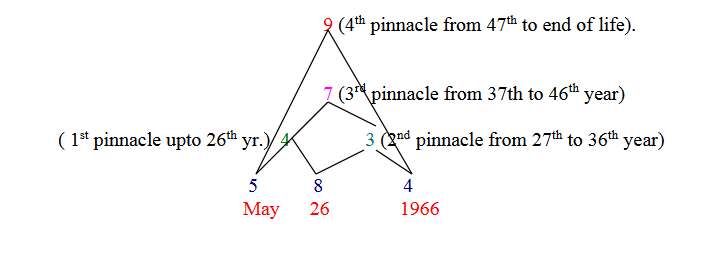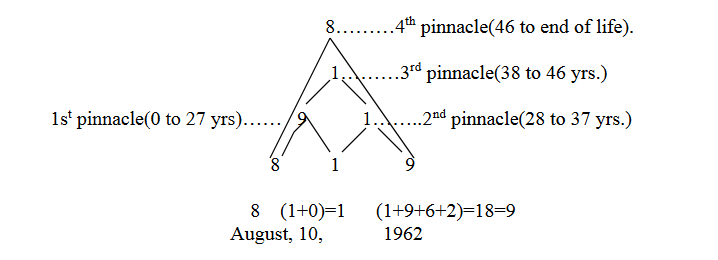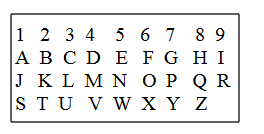error: Content is protected !!

# How to calculate your numbers

1. Fate Number :
Your fate number is arrived at by reducing your birth date to a single digit . If one is born on the 28th of any month his fate number will be 1. For eg. 28=2+8=10=1+0 =(1). In numerology there are only nine numbers,i.e.the lowest number is one and the highest number on reduction is nine. A person born on the 27th will have 9 as his fate number,27=2+7=(9). (Please see table of fate numbers for ready reference).

Example: Ajoy Kumar, born on 10.8.1962.

Now we shall consider only the date of the month to calculate the fate number. Ajoy is born on 10th,so his fate number is 1….. (1+0)= 1.

2. Destiny Number :
The single digit reduction of the sum of your date,month and year of birth(DMY) give the destiny number. For eg. If one is born on 28.11.1986,then his destiny digit would be arrived by addition of the entire DMY and reducing it to a single number.2+8+1+1+1+9+8+6=36=3+6= (9).For one born on 2.2.2000(2+2+2)=6,which shall be his destiny number.

Example: Ajoy Kumar,born on 10.8.1962=1+0+8+1+9+6+2=27=(2+7) =9. So Ajoy Kumar’s destiny number is 9.

3. Karmic Compound Number :
The addition of the forename and surname by using the kabala values(given below),by substituting these numerical values to the alphabets of ones name gives us the compound number.So, 20 +19=(39), which is the compound number arrived at on addition of addition of both the forename and the surname.The compound numbers are not reduced to a single digit unlike the fate numbers and the destiny numbers. (Refer to chapter 6,for the interpretation of your compound number).
Let us see another example: AJOY KUMAR

 A J O Y K U M A R 1 1 7 1 2 6 4 1 2 (1+1+7+1) (2+6+4+5+1) 10+15 =25 COMPOUND NUMBER

4. Good day or bad day?:
To know whether a particular day is good or bad for any important work or purpose,you can check out by the following method:. Add the compound number of your name to the single digit of the day you want to know about,and Add this sum to your fate number,and then check the meaning of the resultant number from chapter 6 on karmic compound numbers. For eg, Ajoy Kumar born on 10th August wants to know how will 27th December be for him.

Substituting his name with Hebrew numerical values given in table A,we find the karmic compound number of Ajoy Kumar as under;

Let us see another example: AJOY KUMAR
 A J O Y K U M A R 1 1 7 1 2 6 4 1 2 (1+1+7+1) (2+6+4+5+1) 10+15 =25 COMPOUND NUMBER

25 Ajoy Kumar’s karmic compound number.
Step 2 : Reduce the compound number to a single digit,so 25=(2+5)=7
Step 3 : To this 7(his reduced name number)add the reduced digit of 9 arrived by adding the day you want to know about, i.e. 27th December(2+7)=9,So,7+9=16. .
Step 4 :Add 16 to his birthday,i.e.10th,so 16 +10=26. .
Now check up the meaning of karmic compound numbers 26 in chapter 6,to see whether 27th December would be lucky for him or not..

5. Personal year :
To find your personal year(annual trends), add your month and day of birth to the present year(also called universal year) for eg.if your birthdate is July,6th1966,and you want to know your personal year for the year 2000;

month day  present year/universal year.
July       6        2000
7    +    6    +    2     =15=(6),which shall be your personal year number for the year 2000. Now let us see another example,Ajay Kumar,born on 10.8.1962,wants to know his personal year in 2001,we would calculate it as August, 10, 2001
8 +1+0+ 2+0+0+1=12
(1+2)=3
So,Ajoy’s personal year in 2001 is 3.

So,if you want to know the general trend for any past,present or future year all you have to do is add the sum of your birth month and date to the year you want to know about,and then read the meaning of the personal year in chapter 13.

6. Personal Day :
To know your personal day add your personal year as shown above to the calendric month and date you want to know about.For eg, Ajoy wants to know what kind of day you will have on 9th August. Now as we have seen above Ajoy’s personal year is 3. Now calendar month August=8 and calendar day =9th,so we add 8+9+ 3(Ajoy’s personal year),so 8+9+3=20=(2+0)=2,which shall be Ajoy’s personal day. The interpretation of Ajoy’s personal day is given in chapter 13.

7. Pinnacles :
To find the pinnacles follow the rules given below:

First pinnacle: Add the digits of the month to the digit of the day. Second pinnacle: Add the digit of the day to the digit of the year. Third pinnacle:Add the digits of the first and the second pinnacle.
Fourth pinnacle:Add together the digit of the month and the year of birth.

For eg: 26th May,1966. May 26, 1966.<

5       8      4 = 17=(8)

Now,subtract the destiny digit 8 from 36,we find the duration of the first pinnacle to be for 28 years.
*For interpretations of the pinnacles see chapter :16.
Each subsequent pinnacle lasts for 9 years.See the following example:Another example; Ajoy Kumar born on August 10, 1962.
His destiny number is 9 as we have seen in method of calculating destiny number above in clause2.So,as per the general rule the destiny number has to be always minused from the fixed digit of 36 to see the influence of his first pinnacle.So 36-9=27.So,Ajoy was under the influence of his first pinnacle number 9 upto his 27th year.Subsequently,the influence of each pinnacle would last for 9 years.8. Heart Desire/Soul urge Number :Let us see another example: AJOY KUMAR
For arriving at one’s heart desire number one has to add all the vowels of both the forename and the surname.For this,Pythagorean numerical values of alphabets given in table B are to be used.

Table B :Pythagorean valuesExample:

AJOY KUMAR(Convert the vowels into numbers using Pythagorean numerical values)
1 6        3 1
(1+6) + (3+1)
7       +     4 = 11(1+1) = 2 is the soul rge number of Ajoy Kumar.

9. Expression Number :
This is derived from the addition of your full name using the Pythagorean values of table B(above)

for example :

 A1 J1 O6 Y7 K2 U3 M4 A1 R9 (1+1+6+7) + (2+3+4+1+9) 15 + 19=34=(3+4)= 7.....EXPRESSION NUMBER

10. Transit of letters :
Transits are derived from the letters of the first name. The physical transit is derived from the first name,mental transit from the middle name and the spiritual tranist from the surname.When there is no middle name,the last name provides both mental and spiritual information.

Example:
Now we shall substitute the Pythagorean values of table B and write those values above Amitabh Mukherjee’s name.

 1 4 9 2 1 2 8 2 4 3 2 8 5 9 1 5 5 Pythagorean Values A M I T A B H B M U K H E R J E E 0 1 2 3 4 5 6 0 1 2 3 4 5 6 7 8 Age 7 8 9 10 11 12 13 9 10 11 12 13 14 15 16 17 14 15 16 17 18 19 20 18 19 20 21 22 23 24 25

As A is the starting alphabet in the name for the letter A it shows that the influence of alphabet A will be dominant for the first years of Amitabh Mukherjee’s life(read chapter 15 for the predictive trend of events/influence of respective years). If Amitabh Mukherjee wants to know what kind of health he would have in his 20th year,he should see the physical transit derived from his first name,i.e.he shall be under influence of the letter H.. If he wants to know his spiritual transit he should employ the same method as shown above only on the surname and find out the influence of the alphabet ruling a particular year.

11. Event Number :
Addition of all the three transit letters of one's full name in any particular year reveals that event number which describes your present situation or trend of events which you are currently experiencing. Now if we want to know Amitabh

Mukherjee's event number for his 12th year we shall see in the above table that in his first name he was under the influence of letter I (9) 9 in his 12th year, in his second name he was under the influence of the letter B (2),and under influence of letter H (8) in his 12th year.
Now we add I+B+H=9+2+8=19=1+9=10 =1(Event umber).

Therefore Amitabh Mukherjee's event number in his 12th year was 1. We then look up the interpretations of event number one to see his current period.

 Physical transit;(first name)(alphabetic values) A1 M4 I9 T2 A1 B2 H8 Age in years:(1stname letters 0A 1M 2M 3M 4M 5I 6I 7I 8I 9I 10I 11I 12I 13I 14T 15T Mental transit: (second name/initial) B2 Spiritual transit(surname)(alphabetic values) M4 U 3 K2 H8 E5 R9 J1 E5 E5 Age in years(surname) 1M 2M 3M 4M 5U 6U 7U 8K 9K 10H 11H 12H 13H 14H 15H